DownloadDocumentationMacromodelling → ABOUT

## USING CIRCUIT DESIGN SOFTWARE TO SIMULATE MECHANICAL COMPONENTS

Goals:

• Joint calculation of electrical and mechanical (non-electrical) parts of a device;
• Use of existent circuit design software with equivalent models of mechanical components;
• Development of the algorithm to obtain models of non-electric objects as equivalent electric circuits for further use in circuit design software.

Simulation of the dynamic processes which take place in modern electronic devices requires a high accuracy of the models used. This circumstance is especially important when simulating heterogeneous by their physical nature devices that is specific for modern microelectromechanical systems (MEMS). However, the most widespread CAD systems use, generally, one-aspect simulation, for instance, Spice for electronic circuit analysis, or Adams for virtual simulation of the complex machines and mechanisms that makes it difficult both getting models of the same accuracy order and joint simulation of the all object.s parts. It should be noted that in the most cases simulation of the object in whole is impossible since heterogeneity of the mathematical model.s bases for the subsystems of different nature that complicates integration of the different CAD software simulation results.

To avoid these problems, it is reasonable to use single software to simulate an object but this requires presentation of the subsystems of different physical nature in the basis of a CAD system chosen. For the mechanical systems, there is a method of electromechanical analogies allowing getting a mathematical model of a mechanical object as an equivalent electrical circuit from a finite-element description. In this case, the accuracy of an equivalent circuit obtained matches the accuracy of a finite-element model.

The requirements of a high simulation accuracy leads to a rather small mesh for the finite-element method that results in the increase of the considered equivalent circuit size and make difficulties for its using as a model. To eliminate this disadvantage, it is possible to use reduction methods based on the Y-∆ transformation. Such reduced equivalent circuits have a number of advantages including compactness of the description and the possibility to formalize the algorithm to obtain them.

Let's consider a method of construction of circuit models of mechanical components and their further use by an example of a problem of finding eigenfrequencies of a beam (Fig. 1). The considered construction represents a beam working on a bending. The left end of the beam is fixed motionlessly, right one is free. Force F is applied to the right end perpendicularly to the beam axe. Conventionally, such construction can be considered as a sensitive element of some sensor, like accelerometer. To the right end of the beam the constant force F=100 N is applied.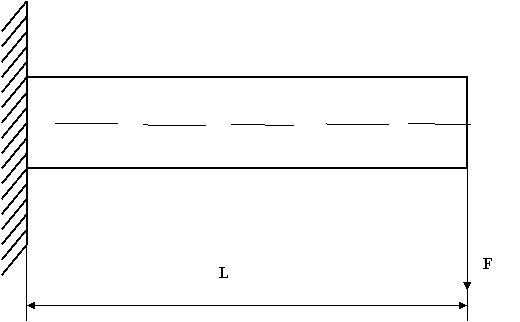Fig. 1. Scheme of fixed beam with three degrees of freedom

Parameters of the construction are: L = 25 mkm; cross-section is orthogonal: height = 3 mkm, width = 2 mkm; material properties: coefficient of elasticity E=2·1011 Pa = 0.2 N/mkm2; Poisson coefficient μ = 0.3; material density ρ = 6·103 kg/m3 = 6·10-9 mg/mkm3.

The following chart describes all the stages needed to simulate a mechanical component:To build a mathematical model, ANSYS Multiphysics v.10.0 developed by ANSYS Inc. was used. For discretization, finite elements have been used, which has two nodes (left/right) with three degrees of freedom in each one: coordinates x, y in a plane of motion and a corresponding beam cross-section's turn angle θ. All the elements have identical length of 0.5 microns. So, the design model consists of 50 beam elements and 51 nodes.

At the next stage, we have to use mor4ansys (http://modelreduction.com/) program to get mass, damping, and stiffness matrices from ANSYS. In the current version of mor4ansys, the two ANSYS files (FULL and EMAT) are used in order to read the system matrices. They could be generated by running such script in ANSYS:

 /solu allsel antype,static eqslv,sparse nsubst,1 wrfull,1 ematwrite,yes solve fini

The mass, damping, and stiffness matrices are written in the Matrix Market format (http://math.nist.gov/MatrixMarket/formats.html). Matrices received from ANSYS are coefficients of equationwhere M, D, and K are mass, damping and stiffness matrices correspondingly; B is an input matrix; C is an output matrix; x is a vector of unknowns, which includes all the degrees of freedom; F is a force vector, and y is a vector of output variables.

Moving to electro-mechanical analogies, we will receive:or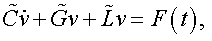where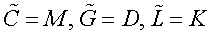are equivalent capacitance, conductance and inductance matrices. The elements of C, G, and L matrices are formed from the elements of M, D and K matrices by formulas:where N is the number of equations for the system given. Using the received C, G, and L matrices, it is possible to proceed to the equivalent circuit (see MM2PS section of this site).

The equivalent circuit of a beam is shown on Fig. 2.Fig. 2. Equivalent circuit of beam with three degrees of freedom

On the basis of the equivalent circuit, by means of RLC reduction program (see RLC section of this site), it is possible to receive a reduced model of the considered mechanical component.

Reduction results as well as simulation by NetALLTED software (see ALLTED section of this site) ones both for equivalent and reduced circuits are shown in Table 1, frequency analysis results on Figs. 3-6.

Table 1. Reduction results

 Source circuit Reduced circuit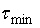- 8*10-4 4*10-4 2*10-4 Number of nodes 154 11 15 27 Number of elements 862 66 94 256 Node reduction, % - 92.8571 90.2597 82.4675 Element reduction, % - 92.3434 89.0951 70.3016 1 peak, MHz 18.745 18.955 18.724 18.788 2 peak, MHz 50.890 52.613 50.458 51.028 3 peak, MHz 97.859 101.75 95.937 97.955 4 peak, MHz 115.49 107.2 112.88 114.93 Maximal error, % - 7.18 2.26 0.48Fig. 3. AFC, PFC of equivalent circuit for fixed uniform beam with three degrees of freedom (non-reduced variant)Fig. 4. AFC, PFC of equivalent circuit for fixed uniform beam with three degrees of freedom (reduced variant,= 8*10-4)Fig. 5. AFC, PFC of equivalent circuit for fixed uniform beam with three degrees of freedom (reduced variant,= 4*10-4)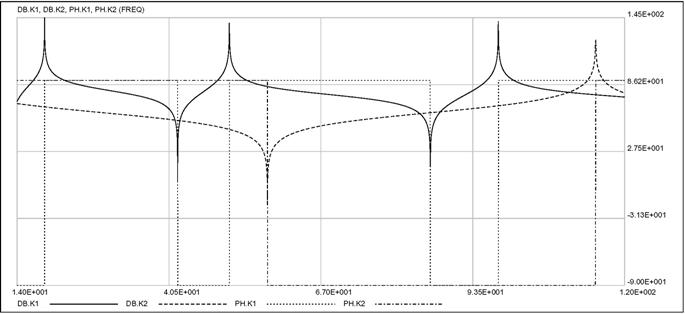Fig. 6. AFC, PFC of equivalent circuit for fixed uniform beam with three degrees of freedom (reduced variant,= 2*10-4)

Conclusions

• The method offered can be used for MEMS calculation, and in the general case it can be expanded on the objects of non-mechanical nature too if their mathematical model can be received in the form of finite-element matrices;
• This approach allows creating the library of mechanical (non-electrical) components and using circuit design software as integrated tools for simulation of electromechanical devices;
• The equivalent circuit obtained does not yield by the accuracy to the mathematical model received by means of the finite-element method;
• Use of the Y-∆ transformation-based reduction allows receiving reduced models with the required accuracy degree.